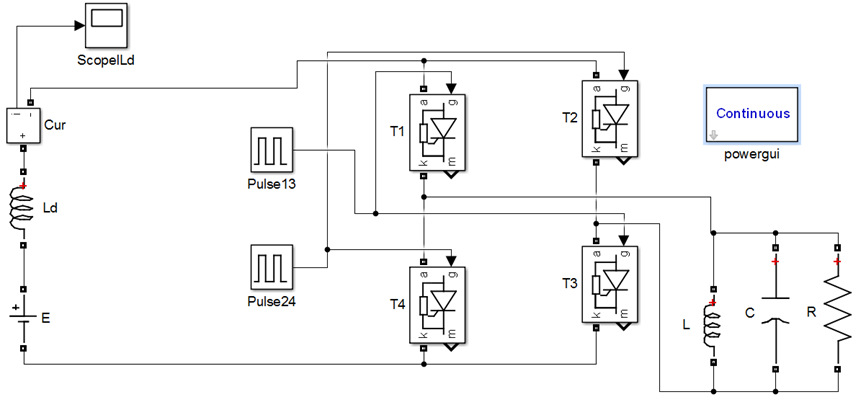# Acceleration of exit to steady-state mode when modeling semiconductor converters

## Authors

• V. G. Yagup Kharkiv National Automobile and Highway University, Ukraine
• K. V. Yagup National Technical University «Kharkiv Polytechnic Institute», Ukraine

## Keywords:

thyristor converter, state variables, difference equations, steady state, visual model

## Abstract

The purpose of the article is to develop a method and algorithm for the accelerated calculation of steady states of thyristor converters using computer models of converters based on the use of the theory of difference equations in the form of recurrent linear relationships for state variables on the boundaries of the converter periods. Methodology. The article is devoted to the solution of the problem of reducing the cost of computer time to achieve the steady state of the thyristor converter. For this, it is proposed to use difference equations, for which the values of the state variables at the limits of the periods of the converter's operation are taken as variables. These values are accumulated during the initial periods of the transient process of the converter, after which the coefficients of the difference equations are calculated, and the following limit values of the state variables are found using the defined difference equations. A program in the algorithmic language of the MATLAB system is presented, which implements the proposed method and algorithm compatible with the visual model of the converter. Results. The theoretical foundations of the proposed method and the area of its applicability are substantiated. Recommendations are presented for determining the number of periods of the flow process that must be calculated for further implementation of the method. An algorithm for forming matrix relations for determining the coefficients of difference equations with respect to the values of state variables at the boundaries of periods is shown. Matrix equations are given that allow calculating the parameters of the steady state. All stages of the algorithm are illustrated with numerical examples. Originality. The method rationally combines all the advantages of visual modeling based on the numerical integration of equations using the method of state variables for the periods of operation of the converter with the analytical solution of the recurrence relations obtained on this basis for the values of state variables at the boundaries of adjacent periods. Practical value. The proposed method makes it possible to reduce by several orders of magnitude the computer time spent on calculating the parameters of the steady-state mode of the converter and, at the same time, to significantly improve the accuracy of these calculations. The practical application of the method is very effective in research and design of thyristor converters of electrical energy parameters.

## Author Biographies

### V. G. Yagup, Kharkiv National Automobile and Highway University

Doctor of Technical Science, Professor

### K. V. Yagup, National Technical University «Kharkiv Polytechnic Institute»

Doctor of Technical Science, Professor

## References

Mohan N., Undeland T.M., Robbins W.P. Power Electronics: Converters, Applications, and Design. John Wiley & Sons, Inc., New York, 2002. 823 p.

Yagup V.G. Automated calculation of thyristor circuits. Kharkov, Vyshcha School Publishing House at KSU, 1986. 160 p. (Rus).

Rajagopalan V. Computer-Aided Analysis of Power Electronic Systems. Marcel Dekker, Inc., New York, 1987. 552 p.

Bakhvalov N.S., Zhidkov N.P., Kobel'kov G.M. Numerical Methods. Moscow, BINOM Publ., 2008. 636 p. (Rus).

Aprille T., Trick T. A computer algorithm to determine the steady-state response of nonlinear oscillators. IEEE Transactions on Circuit Theory, 1972, vol. 19, no. 4, pp. 354-360. doi: https://doi.org/10.1109/TCT.1972.1083500.

Aprille T.J., Trick T.N. Steady-state analysis of nonlinear circuits with periodic inputs. Proceedings of the IEEE, 1972, vol. 60, no. 1, pp. 108-114. doi: https://doi.org/10.1109/PROC.1972.8563.

Moskovko A., Vityaz O. Periodic steady-state analysis of relaxation oscillators using discrete singular convolution method. 2017 IEEE 37th International Conference on Electronics and Nanotechnology (ELNANO), 2017, pp. 506-510. doi: https://doi.org/10.1109/ELNANO.2017.7939803.

Verbiczkij E.V., Romashko V.Y. Application of Difference Equations in Predictive Control Systems for DC-DC Converters. Electronics and Communications, 2012, vol. 17, no. 2, pp. 23-27. doi: https://doi.org/10.20535/2312-1807.2012.17.2.220024.

Mikchalchenko G.Ya., Mulikov D.S. Operation modes of frequency converter with active rectifier. Proceedings of TUSUR University, 2016, vol. 19, no. 2, p. 79-83. (Rus).

Cheng X., Chen Y., Chen X., Zhang B., Qiu D. An extended analytical approach for obtaining the steady-state periodic solutions of SPWM single-phase inverters. 2017 IEEE Energy Conversion Congress and Exposition (ECCE), 2017, pp. 1311-1316. doi: https://doi.org/10.1109/ECCE.2017.8095941.2023-04-23

## How to Cite

Yagup, V. G., & Yagup, K. V. (2023). Acceleration of exit to steady-state mode when modeling semiconductor converters. Electrical Engineering & Electromechanics, (3), 47–51. https://doi.org/10.20998/2074-272X.2023.3.07

## Section

Industrial Electronics